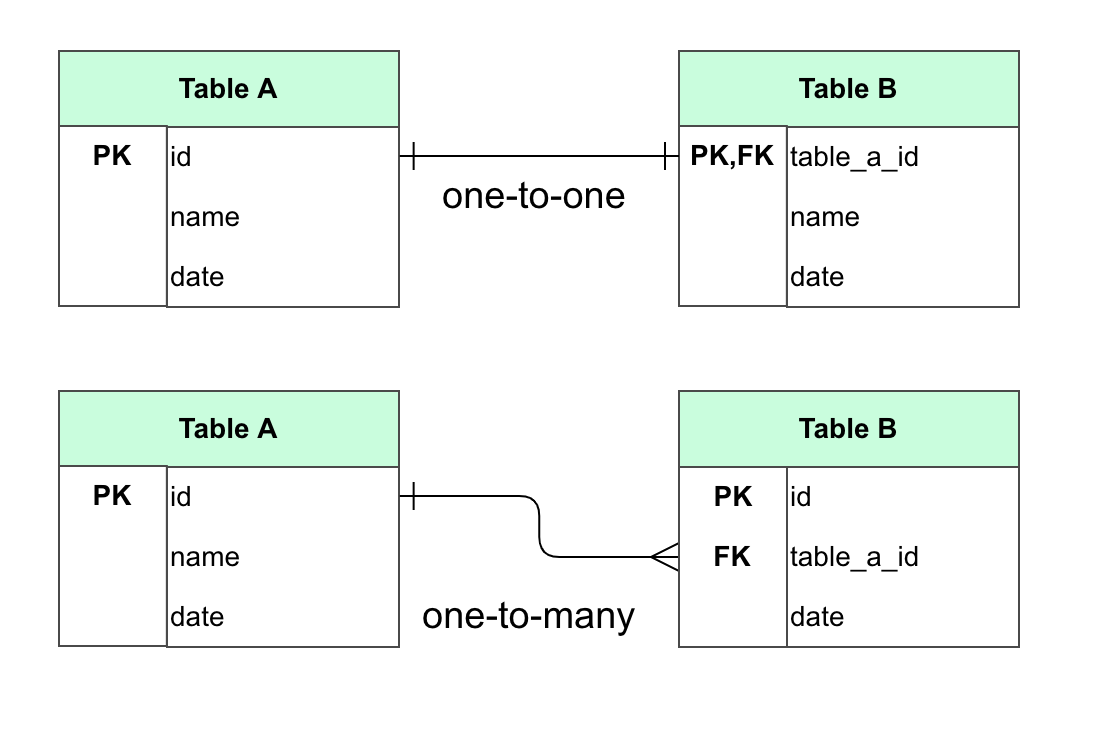1. Learn center
2. Software development
3. Posts
4. Creating Cacoo’s database import autolayout

# Creating Cacoo’s database import autolayout

PostsSoftware developmentCacoo Staff

June 09, 2020

Recently, the Cacoo team released a new feature for our Editor: the database schema importer tool. With this new tool, you can extract metadata about your database tables and import them into the Cacoo Editor as a schema diagram that shows the name of columns and data types you defined on your database. But that’s not all!

The resulting schema diagram also shows the relationships between your database objects, including foreign key constraints that establish dependencies from one table to another. Being able to glance over a graphical representation of these dependencies is essential to grasp the hierarchical structure of your data.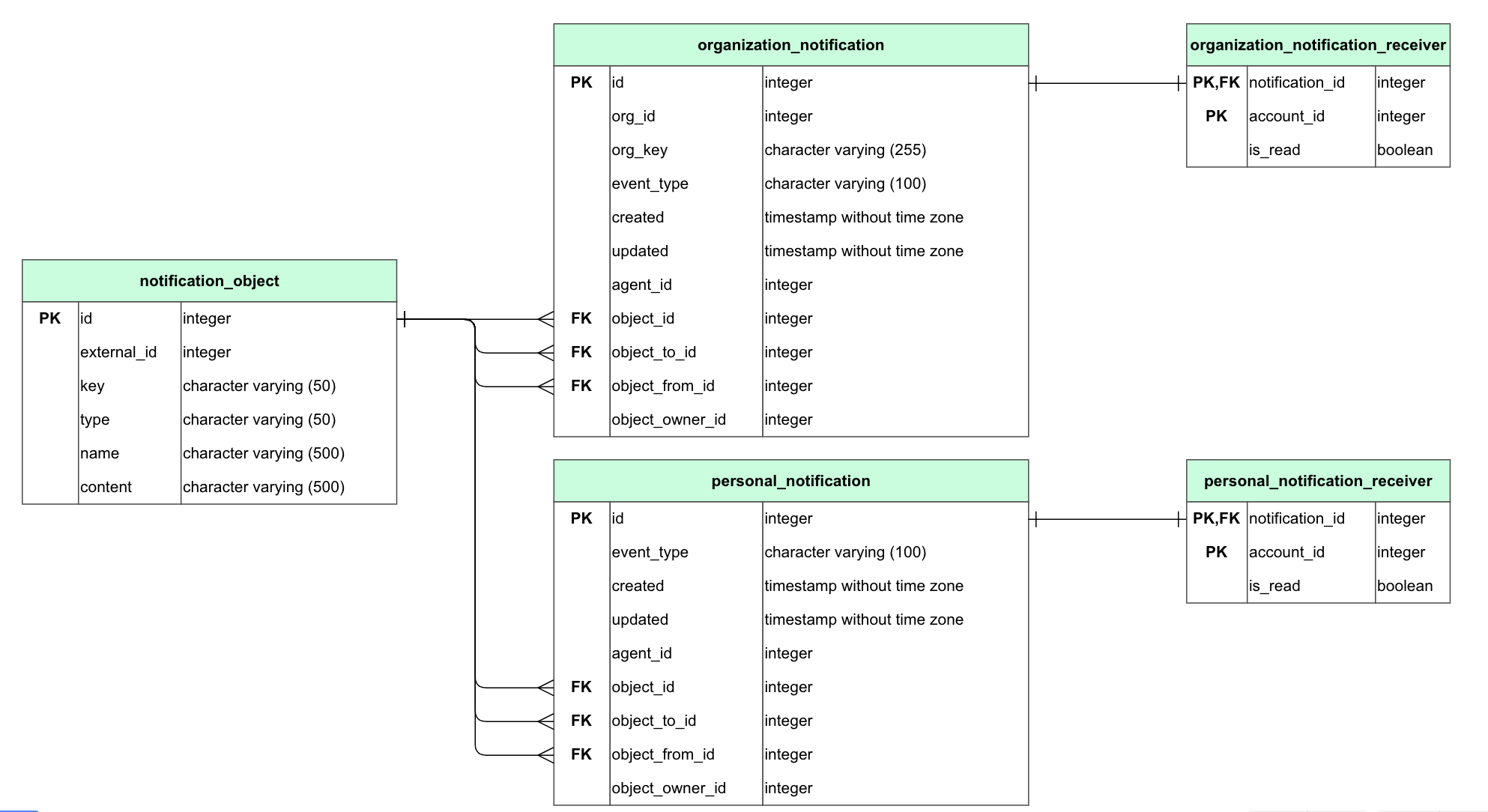Cacoo detects and visualizes foreign key constraints as line connectors and carefully positions all of the shapes on the canvas so that the lines between them are clearly visible.

Here, I’m going to summarize the technique we use to render these nice schema layouts.

## The problem space

Before introducing the autolayout algorithm, it helps to identify a suitable abstraction to model our problem.

Table shapes in our schema diagram are represented as nodes and the foreign key relationships as the connecting lines, also called edges in graph theory. As we place no restrictions on how many nodes and how many edges per node we can have, we can infer that our database schema is indeed a graph. We can go further and say it’s also a directed graph, as foreign key constraints define unidirectional relationships between tables. This is important because drawing undirected graphs instead is a different kind of problem, and we can’t apply the same algorithms to both.

Finally, it’s worth noting that we can’t assume that our directed graph is also acyclic. Even though circular foreign key references might be a sign of poor design, they are possible and might be warranted in some cases. Fortunately, our algorithm can deal with cycles: backward-pointing edges are simply reversed for the duration of the computation, then printed out correctly afterward.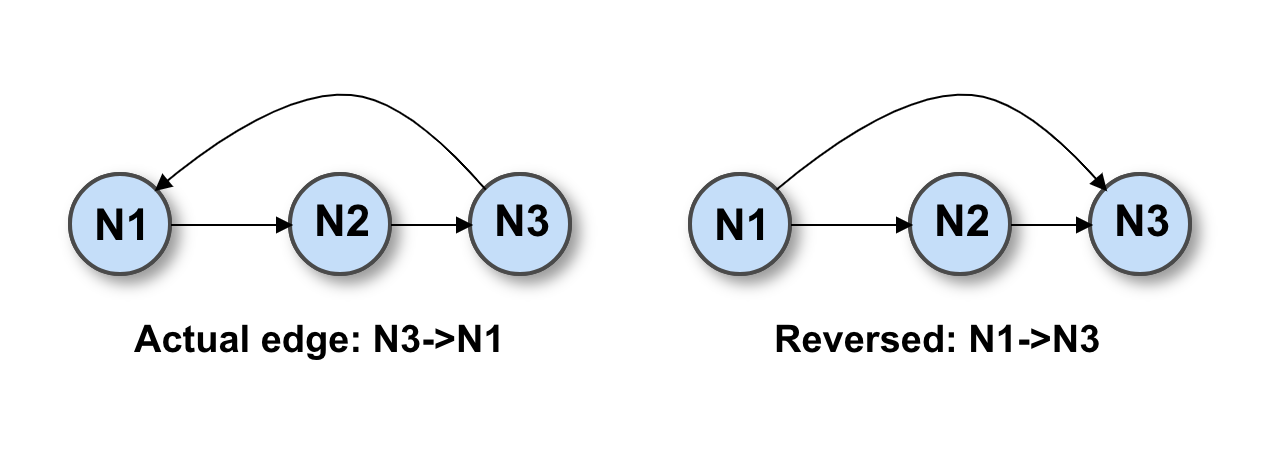So, after you upload your database schema metadata, Cacoo builds a directed graph out of it. Once we have a graph structure, we need to determine the position (x, y) of each shape and the bend points (x, y) of the line connectors. The algorithm we use to do this can be divided for simplicity into a few smaller steps.

## The autolayout algorithm

The four main steps of the directed graph autolayout algorithm are based on the research done by Emden Gansner at Bell Labs. The graphviz software they developed is what powers many libraries out there across a variety of programming languages.

Starting from the directed graph data with nodes and edges as input, the four steps are ranking, ordering, positioning, and routing.

### Ranking

Given a collection of nodes, the ranking step aims to assign each node to a rank based on its incoming and outgoing edges.

Here a rank is basically the level, or layer, on which one or more nodes are aligned. You can imagine them and as a series of parallel rectangular areas. In the picture below, nodes N1, N2, and N3 belong to rank 0; N4 and N5 to rank 1; and N6 and N7 to rank 2.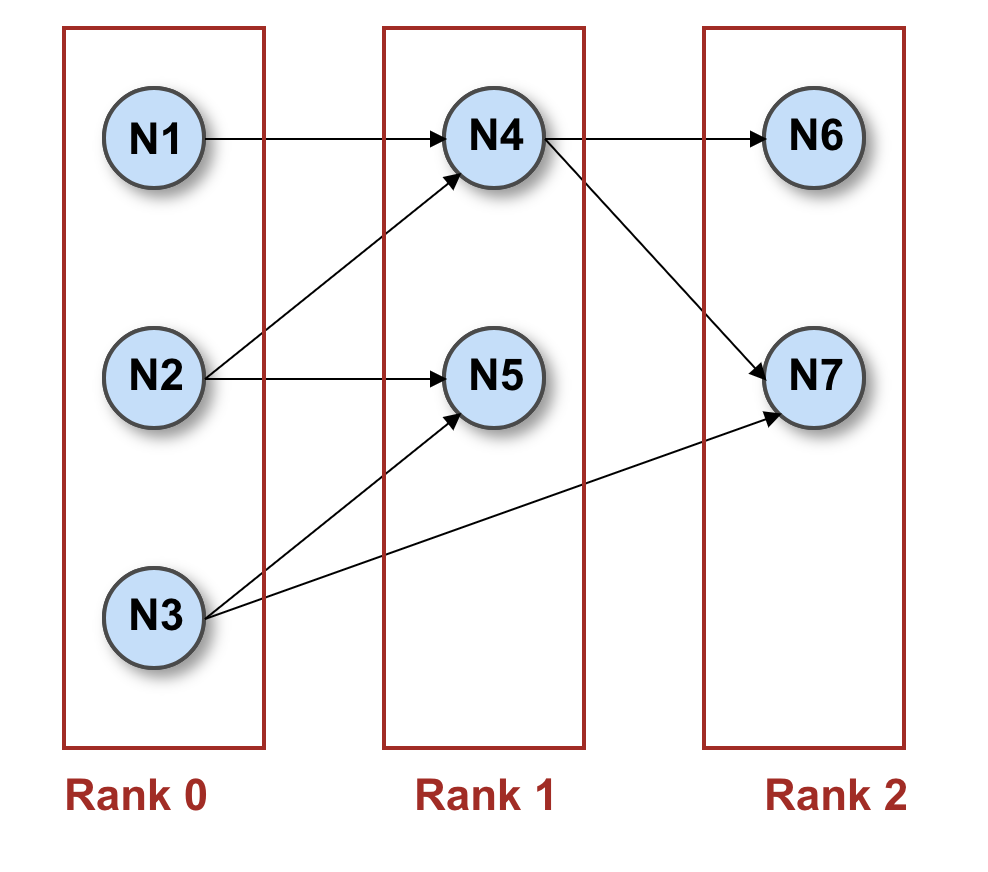Ranks can be laid out horizontally — left to right, as in the picture — or vertically — top to bottom. For schema diagrams, we chose a horizontal rank layout.

The algorithm iterates over the list of nodes and assigns them to ranks so that the length of the edge between two nodes stays above a minimum threshold, usually 1. Length, in this case, means the distance in ranks between two nodes. In the picture, if we call λ(N1) the rank assigned to node N1 and λ(N4) the rank assigned to node N4, the length of the edge N1->N4 is λ(N4)-λ(N1) = 1 – 0 = 1, whereas the edge N3->N7 is λ(N7)-λ(N3) = 2 – 0 = 2, both greater than or equal to 1.

The practical effect of this algorithm is essentially to keep connected nodes as close as possible while avoiding edges along the same rank. Compare the two layouts below, where the node N7 is assigned to rank 2 vs. rank 1.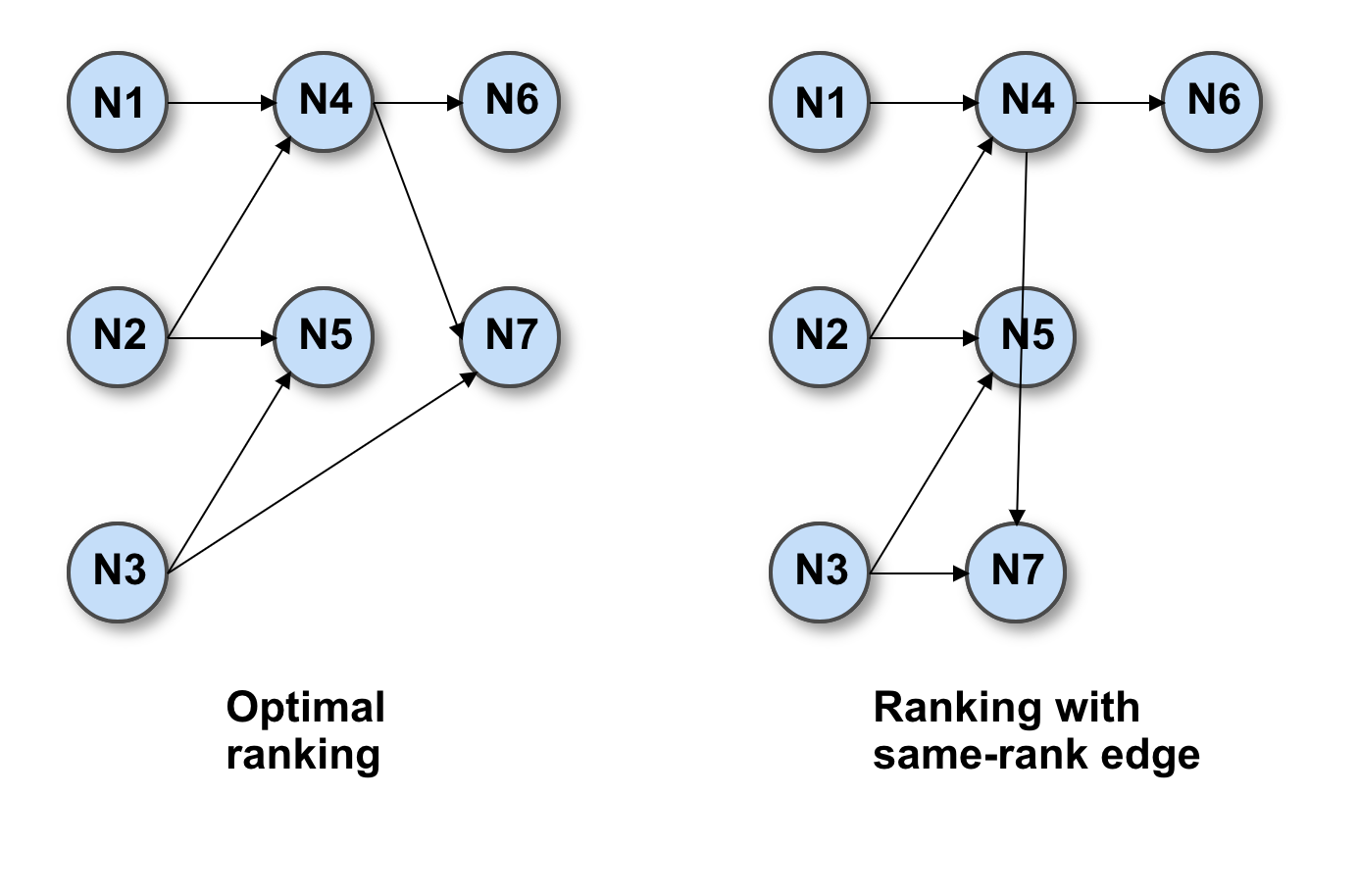This ranking strategy, called network simplex, greatly increases the readability of the final layout and helps to understand the overall flow, especially when there are many nodes and edges.

### Ordering

The second step of our algorithm is ordering the nodes within each rank. In the previous step, we only assigned nodes to ranks, now we must determine in which order they appear on that rank. The purpose of this step is to reduce the number of edge crossings to a minimum. This problem is NP-complete, so the most effective strategies are heuristics.

The basic idea of the algorithm is to iterate over each rank and for each node in that rank to compute its weight based on the position of connected nodes on the next rank. Then we sort nodes by weight.

Commonly, the weight is calculated as the median of the connected nodes, where the numbers we feed to the median function are the current ordinal positions of those nodes.

The following picture illustrates how the algorithm works: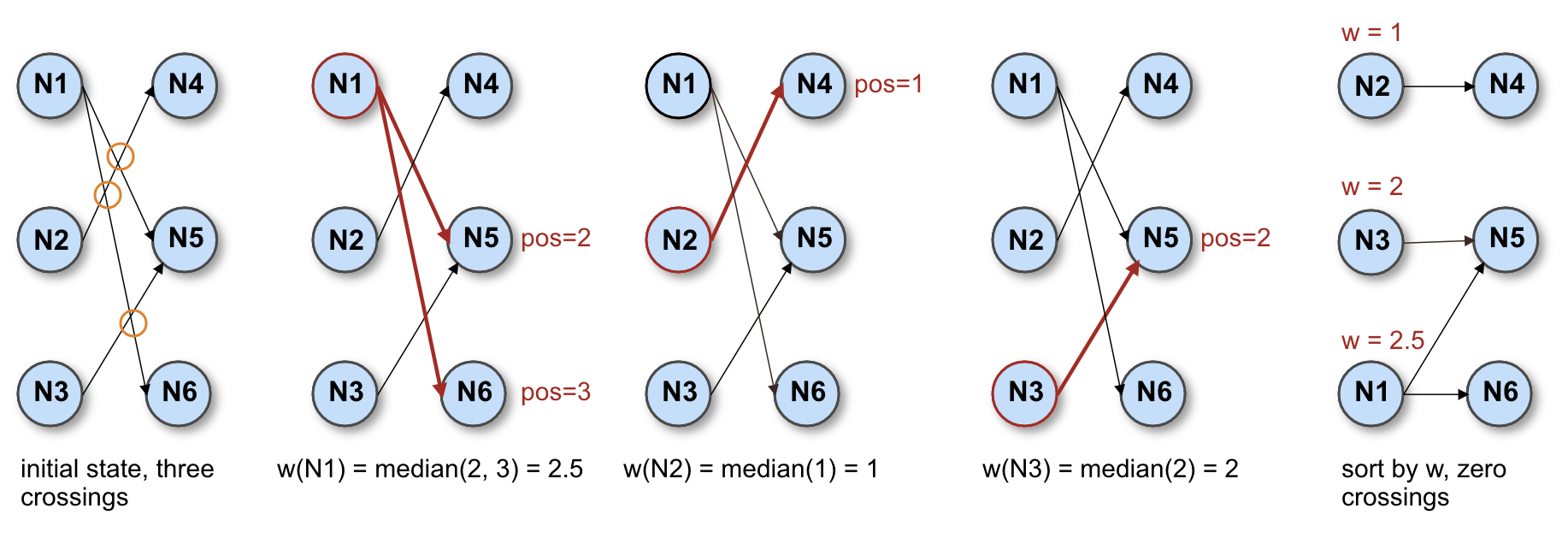In the initial state, we have three line crossings, marked with orange circles. Then we proceed to calculate the weight of N1. As N1 is connected to N5 and N6, which are respectively in position 2 and 3 within their rank, the weight of N1 is median(2,3) = 2.5. We also calculate the weights of N2 and N3 the same way and obtain w(N2)=1 ≥ w(N3)=2 ≥ w(N1)=2.5. After sorting by weight, we end up with zero crossings.

Of course, this is a contrived example. With real-world graphs, you can’t expect to always have zero crossings. In fact, you can’t even expect to find the global optimum; however, these heuristics will give you a close enough approximation to draw decent-looking layouts.

### Positioning

The third step of the algorithm is about assigning actual (X,Y) coordinates to graph nodes. Depending on the orientation of your layout, one of the coordinates is trivial to find. All nodes on the same rank will have the same X value in horizontal layouts, like Cacoo database schemas, or the same Y value on vertical layouts. The algorithm, therefore, is focused on finding the other coordinate.

The procedure involves constructing an auxiliary graph and ranking its nodes with the same network simplex algorithm we used in the first step of the autolayout. The auxiliary graph, which we call G’, comprises the following:

• each node N from the original graph G;
• one node NE for each edge E in G;
• two edges from NE to the nodes connected by E in G;
• one edge for each pair of adjacent same-rank nodes in G.

The following picture gives a visual cue of what an auxiliary graph might look like: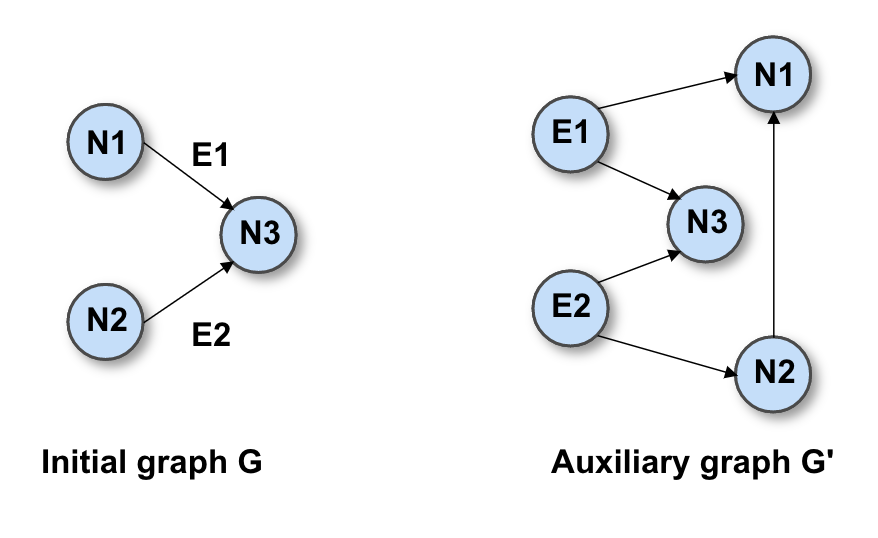This approach works because it can be mathematically shown that the positioning problem in G corresponds to the ranking problem in G’.

Once we have the solution, we set the missing coordinate for each node N in G to its rank in G’.

That final operation might seem weird at first. Why does the rank in G’ become a valid coordinate in G? To easily understand this, remember that in the first autolayout step the goal of the network simplex was essentially to minimize the length of the edges while staying above a certain threshold. In the first step, this threshold was a small constant value. In this step, instead, the threshold is computed. And for nodes that belong to the same rank in G, the computation takes into account the nodes’ actual size and spacing. This produces higher values, and ultimately in G’, these nodes end up in ranks quite far apart. That’s why we can use ranks in G’ as coordinates in G.

### Routing

After we determine the rank, order, and coordinates of our nodes, the final step is to display the line connections between them. Edge routing is the process by which we find the most appropriate start, end, and bend points to draw these lines so that they show up in an orderly fashion and don’t cross other nodes.

The topic is complex enough to warrant its own blog post. For now, I’ll introduce the main concepts behind edge routing.

Intuitively, the goal is to keep edges as short as possible. When two connected nodes belong to adjacent ranks and they are closely aligned, or when there’s only empty space between them, the edge can often be drawn as a straight line. However, when there are other intervening nodes, we require a more sophisticated routing mechanism.

The task is to find the empty region of the diagram in which the edge can be drawn, and then divide it into rectangular areas. These areas, or boxes, are perpendicular to the direction of the layout and are bounded by other nodes and edges.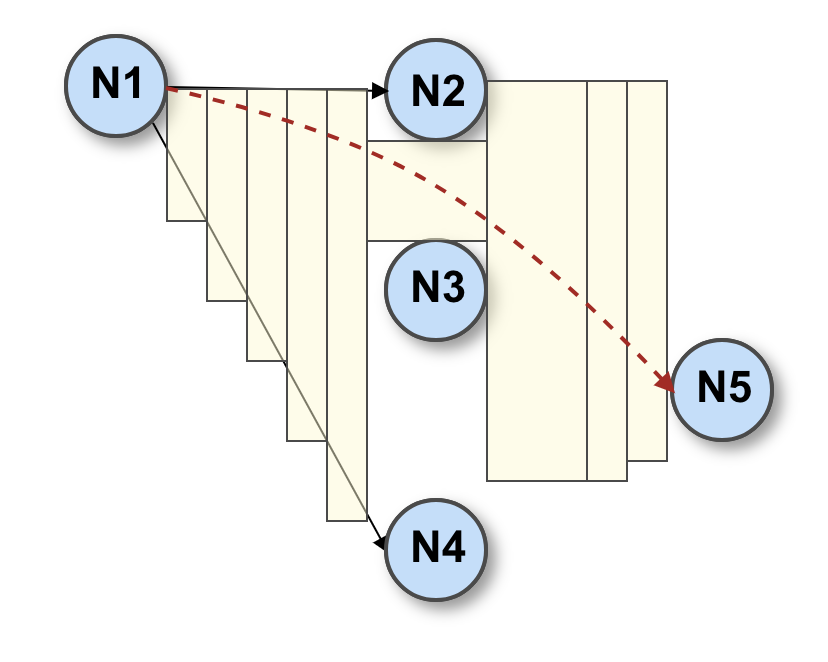In the picture above, a straight line between N1 and N5 might cross over N3. The available space between the start and end nodes is then divided into smaller sections, highlighted in yellow, that can encompass segments of the edge.

Then we proceed to compute the actual piecewise curve that connects the start and endpoints, lying inside the target region. In the case of orthogonal connectors, the algorithm finds the bend points; in the case of curved connectors, it calculates the Bezier splines. In both cases, the goal is to approximate the shortest path and avoid sharp turns or elbows that might worsen the graphic quality of the output.

As a final touch, Cacoo also draws line terminators, or arrowheads, according to the cardinality of the relation between two database tables. When a foreign key column is also a primary key, the arrowheads represent a one-to-one cardinality. Otherwise, they represent a one-to-many cardinality.